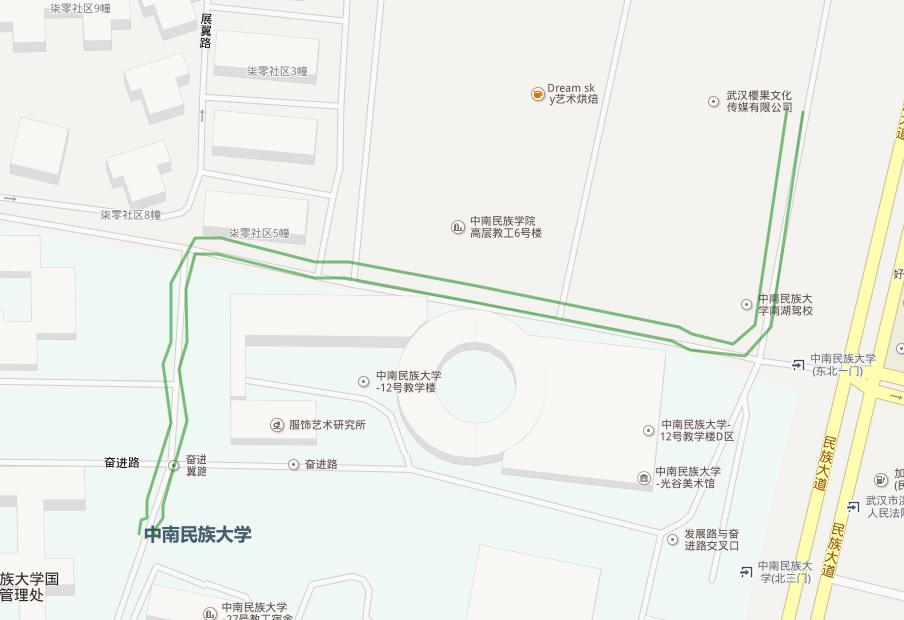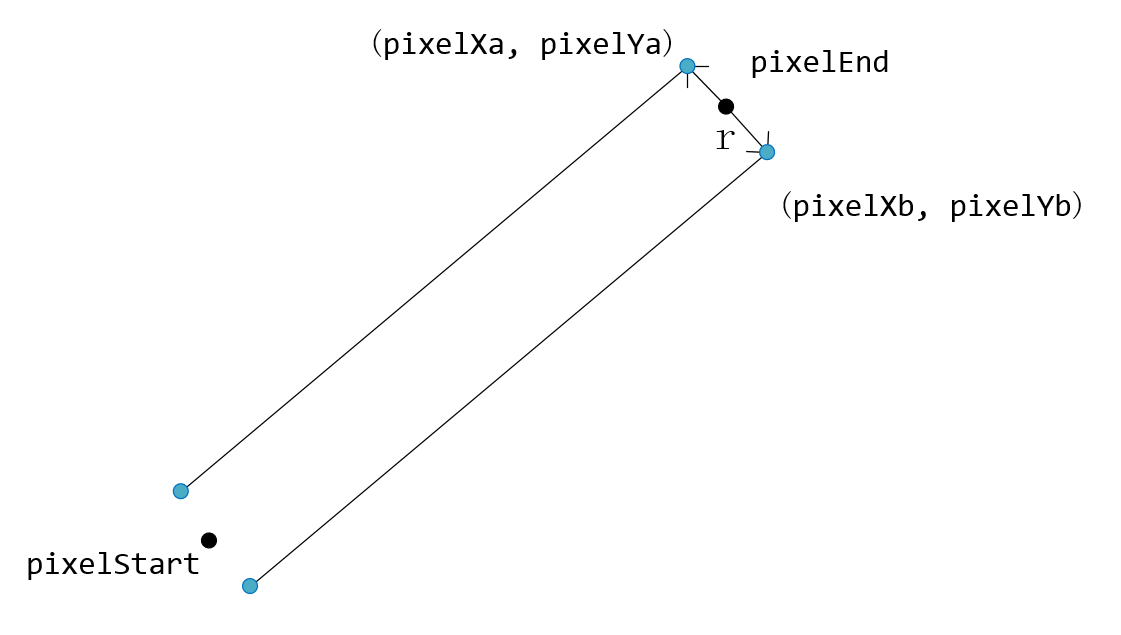• 2018_2019学年高中数学第三章直线与方程3.1.2两条直线平行垂直的判定情境导学素材新人教A版必修2
• 平面内两条线平行条件： 平行线的k斜率相等： l1: y= kx+b; l2: y =kx+c 两条互相垂直直线,其斜率是互为负倒数. 即 k1=-1/k2. 或 k1·k2=-1. 相交点坐标 a为跟随移动的点，c为固定点，move为移动点，求过...
平面内两条线平行条件：

平行线的k斜率相等： l1: y= kx+b;     l2: y =kx+c

两条互相垂直的直线,其斜率是互为负倒数.

即 k1=-1/k2.
或 k1·k2=-1.

相交点坐标

a为跟随移动的点，c为固定点，move为移动点，求过一点d与直线垂直的交点？
ps：
y = kx + b , 已知两点求ac bc所在直线方程
y = -x/k +b2 直线垂线方程

        let k1 = (a.y-c.y)/(a.x- c.x);
let b1 = c.y-(a.y-c.y)/(a.x- c.x)*c.x;
let b2 = move.y + move.x/k1
let d = {};
d.x = (b2/k1 - b1/k1)/(1+(1/Math.pow(k1,2)));
d.y = k1*d.x+b1;

以上方式是也是对已知一固定点c，c两端延伸出两条线段并且垂直，move点跟随鼠标移动，那么未知点有两个a和b以上求出了d，即是a移动后的点新位置坐标那么b怎么求，同理如下：

        // 求 e
let k2 = (b.y-c.y)/(b.x- c.x);
let b3 = c.y-(b.y-c.y)/(b.x- c.x)*c.x;
let b4 = move.y + move.x/k2
let e = {};
e.x = (b4/k2 - b3/k2)/(1+(1/Math.pow(k2,2)));
e.y = k2*e.x+b3;

实践可用靠谱

展开全文自动驾驶 算法
• 根据他人的程序修改的...在此，使用两条平行线表示路线。 1.坐标计算： 2.代码如下： String path = request.getContextPath(); String basePath = request.getScheme() + "://" + request.getServerName
根据他人的程序修改的，原文是如何利用百度地图JSAPI画带箭头的线？
在此，使用两条平行线表示路线。1.坐标计算：2.代码如下：
<%@ page language="java" import="java.util.*" pageEncoding="UTF-8"%>
<%
String path = request.getContextPath();
String basePath = request.getScheme() + "://"
+ request.getServerName() + ":" + request.getServerPort()
+ path + "/";
%>

<!DOCTYPE HTML PUBLIC "-//W3C//DTD HTML 4.01 Transitional//EN">
<html>
<base href="<%=basePath%>">

<title>值班员主界面</title>
<meta http-equiv="Content-Type" content="text/html; charset=utf-8" />
<meta name="viewport" content="initial-scale=1.0, user-scalable=no" />
<style type="text/css">
body,html {
width: 100%;
height: 100%;
margin: 0;
font-family: "微软雅黑";
}

#allmap {
height: 100%;
width: 100%;
}
</style>
<script type="text/javascript"
src="http://api.map.baidu.com/api?v=2.0&ak=Tv58ayKXGwWUzEcg2WoBw1qQrIkVww9B"></script>
<!-- ak表示的是百度地图的key值	-->

<body>
<div id="allmap"></div>
</body>
</html>

<script type="text/javascript">
var routeLine = [
"114.402781,30.498467", "114.402759,30.498304",
"114.402723,30.498086", "114.402683,30.497872",
"114.402647,30.497627", "114.402543,30.49751",
"114.402341,30.497569", "114.402265,30.497592",
"114.401367,30.497751", "114.400917,30.497806",
"114.400792,30.497829", "114.400644,30.497849",
"114.400446,30.497872", "114.400194,30.497915",
"114.400096,30.497934", "114.400028,30.497771",
"114.40001,30.497569", "114.400001,30.497456",
"114.400006,30.49725", "114.399988,30.497106",
"114.399925,30.496946", "114.399898,30.49688",
"114.399884,30.496849", "114.399875,30.496818", ];

var point_local = new BMap.Point(114.399916, 30.493214); //屏幕中心点坐标

// 初始化百度地图API功能
// 创建Map实例,并设置地图允许的最小/大级别(可选)
var map = new BMap.Map("allmap");
var localhost_Icon = new BMap.Icon("images/Point_local.png",
new BMap.Size(32, 49), {anchor: new BMap.Size(16, 48)
});
//设置引用图标的名字以及大小，并设置图片相对于所加的点的位置
map.centerAndZoom(point_local, 16); // 初始化地图,设置中心点坐标(经度，纬度)和地图缩放级别
map.enableScrollWheelZoom(true); // 开启鼠标滚轮缩放

drawRouteLine(routeLine); // 描绘轨迹线路

// 描绘轨迹线路
function drawRouteLine(routeLine) {
if(routeLine.length<2)	return;
var routeLineArray = [];
for ( var i = 0; i < routeLine.length; i++) {
var x = routeLine[i].split(",");
var y = routeLine[i].split(",");
routeLineArray[i] = new BMap.Point(x, y);
}
var polyline = new BMap.Polyline(routeLineArray, {strokeColor:"red", strokeWeight:2, strokeOpacity:0.5}); //创建折线

var linePoint = polyline.getPath(); //线的坐标串

var length = 1;		//管子半径
var LinePointUp=new Array(), LinePointDown=new Array();
for (var i=0; i<linePoint.length; i++) {
var pixelStart;
var pixelEnd;
var PixelArr;

if(i==0){
pixelStart = map.pointToPixel(linePoint[i+1]); //坐标转换为可视区域坐标
pixelEnd = map.pointToPixel(linePoint[i]);
}else {
pixelStart = map.pointToPixel(linePoint[i-1]);
pixelEnd = map.pointToPixel(linePoint[i]);
}
//计算偏差点坐标
PixelArr = getPixel(pixelStart, pixelEnd, length);
var pixelPointa = map.pixelToPoint(new BMap.Pixel(PixelArr,PixelArr));
var pixelPointb = map.pixelToPoint(new BMap.Pixel(PixelArr,PixelArr));
if(i==0){		//第一个点需要交换处理
var pixelPointTem = pixelPointa;
pixelPointa=pixelPointb;
pixelPointb=pixelPointTem;
}
LinePointUp[i] = pixelPointa;
LinePointDown[i] = pixelPointb;
}
var PixelLine1a = new BMap.Polyline(
LinePointUp, {strokeColor:"green", strokeWeight:2, strokeOpacity:0.5});

var PixelLine2b = new BMap.Polyline(
LinePointDown, {strokeColor:"green", strokeWeight:2, strokeOpacity:0.5});
}

function getPixel(pixelStart, pixelEnd, length){
var r = length; 	//管子半径
var delta = 0; 		//主线斜率，垂直时无斜率
var param = 0; 		//代码简洁考虑
var pixelXa, pixelYa, pixelXb, pixelYb;
//计算偏差点坐标
if (pixelEnd.x - pixelStart.x == 0) { //斜率不存在是时
if (pixelEnd.y > pixelStart.y) {
pixelXa = pixelEnd.x-r;
pixelXb = pixelEnd.x+r;
}else{
pixelXa = pixelEnd.x+r;
pixelXb = pixelEnd.x-r;
}
pixelYa = pixelEnd.y;	pixelYb = pixelEnd.y;
} else { //斜率存在时
delta = (pixelEnd.y - pixelStart.y)/(pixelEnd.x-pixelStart.x);	//斜率
param = Math.sqrt(delta * delta + 1);
//已知直角三角形两个点坐标及其中一个角，求另外一个点坐标算法
if (pixelEnd.x > pixelStart.x) {	//第一、四象限
pixelXa = pixelEnd.x-r*delta/param;		pixelYa = pixelEnd.y+r/param;
pixelXb = pixelEnd.x+r*delta/param;		pixelYb = pixelEnd.y-r/param;
}else{		//第二、三象限
pixelXa = pixelEnd.x+r*delta/param;		pixelYa = pixelEnd.y-r/param;
pixelXb = pixelEnd.x-r*delta/param;		pixelYb = pixelEnd.y+r/param;
}
}
return [pixelXa,pixelYa, pixelXb, pixelYb];
}

</script>
现在的问题：在拐角的转折做的不好，特别是在外角部分。


展开全文• 所以说,如果两条直线平行,则它们的斜率相等【是错误的】 反过来:若两直线斜率相等,则这两条直线平行【是正确的】 第二步：思路： 证明两条直线平行，肯定是证明斜率相同比较容易，因为根据坐标求斜率比较简单，但是...
第一步：原理：
1.若两条直线斜率存在,则:斜率相等,则这两直线平行;
2.若两条直线斜率都不存在,则这两条直线也平行.所以说,如果两条直线平行,则它们的斜率相等【是错误的】 反过来:若两直线斜率相等,则这两条直线平行【是正确的】
第二步：思路：
证明两条直线平行，肯定是证明斜率相同比较容易，因为根据坐标求斜率比较简单，但是需要注意的是，当直线平行于y轴的时候斜率是不存在的，所以在写代码的时候要考虑到这一点
第三步：代码：
public static void parallel(double x1,double y1,double x2,double y2,double x3,double y3,double x4,double y4){
//这个判断条件是为了避免有一条直线平行于y轴，因为他们此时斜率无穷大，但是如果他们都平行y轴，说明他们也是平行的
if(x1==x2 || x3==x4){
if(x1==x2&&x3==x4){
System.out.println("两直线平行");
}
}else{
double p1 = (y2-y1)/(x2-x1);
double p2 = (y4-y3)/(x4-x3);
if(p1 == p2){
System.out.println("两直线平行");
}
}
}

第四步：拓展：
思考：当判断三点坐标是不是在一条直线上的时候，这个是不是也可以用到？
很明显是可以的，话不多说上代码：
public static void getSlope(double x1,double y1,double x2,double y2,double x3,double y3){
//这个判断条件是为了避免有一条直线平行于y轴，因为他们此时斜率无穷大，但是如果他们都平行y轴，说明他们也是平行的
if(x1==x2 || x2==x3){
if(x1==x2&&x2==x3){
System.out.println("该三点在一条直线上");
}
}else{
double p1 = (y2-y1)/(x2-x1);
double p2 = (y2-y3)/(x2-x3);
if(p1 == p2){
System.out.println("该三点在一条直线上");
}
}
}

ok，还有什么证明会用到斜率相同呢，一起探讨探讨吧


展开全文java
• 总结平行线的判定课后测试题1、下列说法正确的有〔 〕①不相交的两条直线平行线; ②在同一平面内,不相交的两条线段平行③过一点有且只有一条直线与已知直线平行; ④若a∥b,b∥c,则a与c不相交.A.1个 B.2个 C.3个 D....
总结平行线的判定课后测试题1、下列说法正确的有〔 〕①不相交的两条直线是平行线; ②在同一平面内,不相交的两条线段平行③过一点有且只有一条直线与已知直线平行; ④若a∥b,b∥c,则a与c不相交.A.1个 B.2个 C.3个 D.4个2、在同一平面内,两条不重合直线的位置关系可能是〔 〕A.平行或相交 B.垂直或相交 C.垂直或平行 D.平行、垂直或相交3.如图3所示,能判断AB∥CE的条件是( )A.∠A=∠ACE B.∠A=∠ECD C.∠B=∠BCA D.∠B=∠ACE4.下列说法错误的是( )A.同位角不一定相等 B.内错角都相等C.同旁内角可能相等 D.同旁内角互补,两直线平行5.不相邻的两个直角,如果它们有一边在同一直线上,那么另一边相互( )A.平行 B.垂直 C.平行或垂直 D.平行或垂直或相交6、在同一平面内的.三条直线，若其中有且只有两条直线互相平行，则它们交点的个数是〔 〕A、0个 B、1个 C、2个 D、3个以上就是数学网为大家整理的平行线的判定课后测试题：精选初一下册数学，大家还满意吗?希望对大家有所帮助!【总结平行线的判定课后测试题】相关文章：
展开全文• 一、问题   已知两条直线l1(x1,y1,x2,y2)l_1(x_1,y_1,x_2,y_2)l1...若平行，计算出两条直线间的平行距离。 二、分析 y−y1y2−y1=x−x1x2−x1⇒{ax+by+c=0a=−(y2−y1)b=x2−x1c=(y2−y1)x1−(x2−x1)y1k=−ab \fra.opencv
• ''' 线1 ''' 线2 ''' 返回是否平行 Public Shared Function IsParallelLine(ByVal curve1 As Curve, ByVal curve2 As Curve) As Boolean If curve1.GetType.ToString <> curve2.GetType.ToString Then Return False...
• 利用python实现判断两条直线是否平行，若相交，输出交点。 自己输入四个点坐标，代表两条直线。p1(x1,y1)，p2(x2,y2)，p3(x3,y3)，p4(x4,y4)。根据判断交点p是否存在来判断两直线位置关系。 // x1,y1,x2,y2,x3,y3,x4...python 经验分享
• 除了根据目视判别来绘制点、线、面图形外，还需要根据特定的数字化条件进行快速、有效的数据绘制，如根据坐标绘制直线端点、定长直线平行线垂直线等。本实验讲述了数字化线要素时绘制自定义直线、定长直线平行...arcgis
• 垂直与平行教学设计及分析 教学内容人教版小学数学第七册 教科书第64-65页 教学目标 知识目标帮助学生初步理解垂直与平行是同一平面内两条直线的两种位置关系初步认识垂线和平行线 技能目标培养学生的空间观念及想象...
• 下面是修正后的/// /// 计算两条直线的交点/// /// L1的点1坐标/// L1的点2坐标/// L2的点1坐标/// L2的点2坐标/// public static PointF GetIntersection(PointF lineFirstStar, PointF lineFirstEnd, PointF ...
• 线的方向向量： 例：墙的方向向量 Line wallLine = (wall.Location as LocationCurve).Curve as Line;...判断个向量平行： faceDir.IsAlmostEqualTo(wallDir); //or 向量相反 faceDir.IsAlmostEqualTo(-wallDir);
• 利用eigen库内矩阵运算函数，写了LinesPositionRelationship3D类。实现了确定三维空间任意两条直线位置关系并获得在平行和交错条件下的两直线距离的功能。该类是在确定空间两圆柱轴线关系下的副产品。
• 已知向量a(x1,y1,z1)、b(x2,y2,z2) 判断垂直，需要点积为0。公式为： a*b=x1x2+y1y2+z1z2=0 在Revit中，
• 刘亚琼地区： 河南省 - 许昌市 - 襄城县学校：...了解平行线的概念、平面内两条直线的相交和平行的两种位置关系, 知道平行公理以及平行公理的推论.3.会用符号语方表示平行公理推论, 会用三角尺和直尺过已知直线外...
• 初中三年数学几何公式、定理梳理，今天分享给大家，家长可以为孩子转发，让孩子的几何学习更方便些。1.过两点有且只有一条直线2.两点之间线段最短3....如果两条直线都和第三条直线平行，这两条直线也互相平行9....
• ## 异面直线垂直

千次阅读 2017-08-02 12:04:08
证明异面直线垂直，标准的方法通常是构造一个跟其中一条直线垂直的、经过另一直线的平面。然而，我发现用几何的方法费力而且白搭，只是跟定理搭上界、并不能简化问题。解析方法的优势非常明显。如果用：个向量...
• 00. 目录 文章目录00. 目录01. PolyWorks概述02. PCB字符机平行度03. PCB字符机垂直度04. 导出测试报告05....01. PolyWorks概述 ...前提条件：已经采集到需要测试的两条直线 2.1 创建笛卡尔坐标系 2.2 选择创建笛激光跟踪仪 精密测量 API
• 功能需求：计算图像中的直线，并过滤掉水平和垂直直线。源码#include #include #include <string>#include "opencv2/core/core.hpp" #include "opencv2/imgproc/imgproc.hpp" #include "opencv2/highgui/highgui...opencv
• 该算法根据直线特征和其在摄像机图像上投影直线共面性质建立模型，利用单位四元素法简化了投影模型变量，进而在仅知被测物体上两条直线相互垂直的信息，利用两条垂线投影在两个摄像机图像上四条直线图像，求解出被测...
• 2、若都存在，看两直线是否垂直； 3、若两直线斜率都存在且不垂直用公式求。 求解第一问： 当直线L1与L2相交但不垂直时，在θ和π-θ中有且仅有一个角是锐角，我们把其中的锐角叫两直线的夹角。 tan(θ1) ...
• 两角有一条公共边,他们的另一边互为反向延长线,具有这种关系的角... 两条直线相交角为90度,那么这两条线互相垂直.其中的一条直线叫做另一条直线的垂线,它们的焦点叫做垂足. 过一点有且只有一条直线与已知直线垂直. ...
• // 得到直线的tan斜率 double GetTanOfLine( LINE line ) { double x_dis = line.pt0.x - line.pt1.x; if ( x_dis == 0.0 ) return 10e9; return (line.pt0.y - line.pt1.y) / x_...// 得到两条平行线之间的距离 doualgorithm c++
• 相交 一般情况 特殊情况 对顶角相等 邻补角互补 垂直 垂线段最短 点到直线的距离 1 2 3 4 垂线的性质 本章知识结构 两条直线的位置关系 平行 平行线的性质 平行线的判定 一概念 1在同一平面内两条直线的位置关系有 ...
• a和b分别是在一个平面上不平行两条直线上，那么显而易见与a和b所在直线垂直程度最高的就是与a和b俩俩垂直的竖线，也就是叉积axb方向平行的直线。两条直线可以用叉积，那么多于两条的情况呢？想象如果又有了一条...
• 两线段对应的直线为ls、lt，采用向量表示法如下： ls=s0+cs⋅u⃗ lt=t0+ct⋅v⃗ 当0≤cs、ct≤1时，上述式表达 设最短距离点分别为sj、tj，则有 sj=s0+sc⋅u⃗ tj=t0+sc⋅v⃗ 其...
• echarts 添加一条平行于x轴的标示线   echarts 添加一条平行于x轴的标示线是markline下面的属性： data : [ [ {name: '标线1起点', value: 100, x: 50, y: 20}, {name: '标线1终点', x: 150, y: 120} ], [ ...
• 在一个2维平面中有两直线(点到点、(点到点，这两条直线的交点用行列式表示如下： 行列式可变形写作： 该交点是由4个点、两两一组确定的线段所在位置的直线的交点 根据贝塞尔参数可以将两直线定义为： 其中...opencv
• 思路：我们先判断这两条直线是否平行平行 再去判断是否重合 若不平行 算交点 具体实现：对于平行 我们直接判断v1,v2的叉积（v1,v2是来表示L1,L2的向量）是否为0 对于重合 我们把l1上的端点p1代入l2 判断叉积 ......